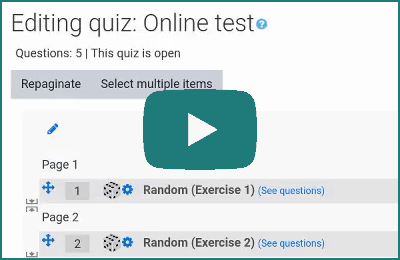Want to share your content on R-bloggers? click here if you have a blog, or here if you don't.

Step-by-step tutorials for generating, importing, and customizing online tests and quizzes using exams2moodle() and exams2openolat() in R/exams.## Motivation

E-learning resources such as online tests and quizzes or more formal e-exams are very useful in a variety of settings: formative vs. summative assessments; in-class vs. distance learning; synchronous vs. asynchronous; small vs. large groups of students. Some typical examples are outlined here.• Short tests/quizzes conducted in-class (synchronously) as a quick assessment of content the students had to prepare before class. In a flipped classroom approach the test/quiz might also be conducted after a collaboration phase in class.
• Asynchronous online tests that students can do in their own time (e.g., over several days) to obtain (incentivized) feedback regarding their learning progress. Such tests might follow any form of content delivery, be it classical lectures or video screencasts or some other approach.
• Synchronous e-exams conducted in-class or remotely (e.g., coupled with a safe exam browser) as a summative assessment at the end of a course.

R/exams can support these scenarios by creating a sufficiently large number of randomized versions of dynamic exercises that can subsequently be imported into a learning management system (LMS). The actual quiz/test/exam is then conducted in the LMS only, i.e., without the need to have R running in the background, because all exercises and corresponding solutions have been pre-computed and stored in the LMS. Popular LMS include the open-source systems Moodle, Canvas, OpenOLAT, or Ilias or the commerical Blackboard system. R/exams provides suitable interfaces for all of these but the capabilities differ somewhat between the LMS. In the following we focus on Moodle and OpenOLAT, both of which provide very flexible and powerful assessment modules.

## Creation in R/exams

Just like for other R/exams interfaces the starting point is putting together a vector or list of (potentially) dynamic exercises in R. From these exercises – in either R/Markdown or R/LaTeX format – a number of random replications can be drawn using either `exams2moodle()` or `exams2openolat()`, respectively. Both interfaces support all R/exams exercise types: single-choice (`schoice`), multiple-choice (`mchoice`), numeric (`num`), string (`string`), or combinations of these (`cloze`). See the First Steps tutorial for more details.Here, we use a collection of exercise templates that are all shipped within the R/exams package and that cover a broad range of different question types as well as different randomyly-generated content (shuffling, random parameters, R output, graphics, simulated data sets).

swisscapital `schoice` Knowledge quiz question with basic shuffling
deriv `num` Computing the derivative of a function with randomized parameters
ttest `mchoice` Interpretation of R output from `t.test()`
boxplots `mchoice` Interpretation of parallel boxplots
function `string` Knowledge quiz question where the answer is the name of an R function
lm `cloze` Conducting a simple linear regression based on a randomly-generated CSV file
fourfold2 `cloze` Completing a fourfold table based on verbal description with randomized parameters

First, we load the `exams` package and define a vector with all exercise `.Rmd` file names.

```library("exams")
elearn_exam <- c("swisscapital.Rmd", "deriv.Rmd", "ttest.Rmd",
"boxplots.Rmd", "function.Rmd", "lm.Rmd", "fourfold2.Rmd")```

Alternatively, the corresponding `.Rnw` files could be used, yielding virtually identical output.

Second, we generate a Moodle XML file with 3 random replications of each of the exercises.

```set.seed(2020-03-15)
exams2moodle(elearn_exam, n = 3, name = "R-exams")```

This yields the file R-exams.xml that can be imported into Moodle.

Analogously, a ZIP archive containing QTI 2.1 XML files (Question & Test Interoperability standard) for import into OpenOLAT.

```set.seed(2020-03-15)
rxm <- exams2openolat(elearn_exam, n = 3, name = "R-exams")```

The resulting output file is R-exams.zip.

Moreover, to show that the object returned within R can also be useful we have assigned the output of `exams2openolat()` to an object `rxm`. This is not necessary but inspecting this object might be helpful when developing and testing new exercises. In particular, we can easily extract the meta-information regarding the correct answers in all randomly generated exercises.

```exams_metainfo(rxm)
##
## exam1
##     1. Swiss Capital: 4
##     2. derivative exp: 38.72 (38.71--38.73)
##     3. 2-sample t-test: 1, 2, 5
##     4. Parallel boxplots: 2, 4, 5
##     5. R functions: lm
##     6. Linear regression: FALSE, FALSE, TRUE | -0.861
##     7. fourfold: 4.44 | 19.74 | 1.56 | 74.26 | 24.18 | 75.82 | 6 | 94 | 100
##
## exam2
##     1. Swiss Capital: 4
##     2. derivative exp: 2 (1.99--2.01)
##     3. 2-sample t-test: 3
##     4. Parallel boxplots: 2, 3, 4
##     5. R functions: vcov
##     6. Linear regression: FALSE, TRUE, FALSE | 0.531
##     7. fourfold: 5.76 | 23.92 | 2.24 | 68.08 | 29.68 | 70.32 | 8 | 92 | 100
##
## exam3
##     1. Swiss Capital: 2
##     2. derivative exp: 2.05 (2.04--2.06)
##     3. 2-sample t-test: 2, 3
##     4. Parallel boxplots: 2, 4, 5
##     5. R functions: glm
##     6. Linear regression: TRUE, FALSE, FALSE | 0.024
##     7. fourfold: 6.5 | 22.5 | 3.5 | 67.5 | 29 | 71 | 10 | 90 | 100
```

## Import into Moodle and OpenOLAT

Finally, the output files generated above can be imported into the Moodle and OpenOLAT learning management system, respectively. In Moodle the random exercises are imported into a question bank based on which a quiz with randomly-selected questions can be constructed. In OpenOLAT the import directly yields a test learning resource that can then be embedded in a course.### Moodle import

A step-by-step video guide to importing and customizing the quiz in Moodle is available on YouTube at https://www.youtube.com/watch?v=5K9hrE3YkPs.### OpenOLAT import

A step-by-step video guide to importing and customizing the test in OpenOLAT is available on YouTube at https://www.youtube.com/watch?v=1ZhdmoDtUSA.